UnboundEd Mathematics Guide

# Geometry: Unbound A Guide to Grade 8 Mathematics Standards

?
What's in a Content Guide and how do I use it?
Get answers to all your Content Guide questions, including what's in each part and how they can be used in your role at your school
View FAQs

8.G.A | Understand congruence and similarity using physical models, transparencies,

or geometry software.

8.G.B | Understand and apply the Pythagorean Theorem.

Welcome to the UnboundEd Mathematics Guide series! These guides are designed to explain what new, high standards for mathematics say about what students should learn in each grade, and what they mean for curriculum and instruction. This guide, the first for Grade 8, includes three parts. The first part gives a “tour” of the standards in the first two clusters of the Geometry domain (dealing with congruence, similarity and the Pythagorean Theorem) using freely available online resources that you can use or adapt for your class. The second part shows how these standards relate to other concepts in Grade 8. And the third part explains where congruence, similarity and the Pythagorean Theorem are situated in the progression of learning from the elementary grades to high school.

# Part 1: What do these standards say?

## Congruence and similarity

Congruence and similarity are also a great way to start the year because they involve a “hands-on” approach through transformations, making them accessible to any student with a basic understanding of lines and angles. Moreover, the standards involving congruence and similarity feed directly into the high school geometry standards (particularly the Congruence and Similarity & Right Triangles domains), so they’re important for students’ future success. So if you’re wondering where to start your year, congruence and similarity are a solid bet.

In Grade 8, the standards dealing with congruence and similarity are grouped together into one cluster (called 8.G.A, since it’s the first cluster of geometry standards in the grade). Despite the fact that it contains only five standards, this cluster manages to bring together a number of mathematical ideas, including three that aren’t often seen as related: congruence and similarity, transformations, and angle relationships. Let’s take a look at what these standards say, and then we’ll examine each one more closely.

8.G.A | Understand congruence and similarity using physical models, transparencies, or geometry software.

 8.G.A.1 Verify experimentally the properties of rotations, reflections, and translations: 8.G.A.1.A Lines are taken to lines, and line segments to line segments of the same length. 8.G.A.1.B Angles are taken to angles of the same measure. 8.G.A.1.C Parallel lines are taken to parallel lines. 8.G.A.2 Understand that a two-dimensional figure is congruent to another if the second can be obtained from the first by a sequence of rotations, reflections, and translations; given two congruent figures, describe a sequence that exhibits the congruence between them. 8.G.A.3 Describe the effect of dilations, translations, rotations, and reflections on two-dimensional figures using coordinates. 8.G.A.4 Understand that a two-dimensional figure is similar to another if the second can be obtained from the first by a sequence of rotations, reflections, translations, and dilations; given two similar two-dimensional figures, describe a sequence that exhibits the similarity between them. 8.G.A.5 Use informal arguments to establish facts about the angle sum and exterior angle of triangles, about the angles created when parallel lines are cut by a transversal, and the angle-angle criterion for similarity of triangles. For example, arrange three copies of the same triangle so that the sum of the three angles appears to form a line, and give an argument in terms of transversals why this is so.

The order of the standards doesn’t indicate the order in which they have to be taught. Standards are only a set of expectations for what students should know and be able to do by the end of each year; they don’t prescribe an exact sequence or curriculum. In this case, it makes sense to first introduce students to the properties of reflections, translations, and rotations (8.G.A.1), and then to use these transformations to establish the concept of congruence (8.G.A.2). From there, students should be ready to take on dilations and the concept of similarity (8.G.A.4). Solving problems with transformations on the coordinate plane (8.G.A.3) can be integrated alongside work with these first three standards, and applications of transformations (8.G.A.5) might also be taught in conjunction with other work, or they might be saved for last.

Before we begin working our way through these standards, let’s pause for a moment and consider why they’re so important. In the past, geometry in Grades K-8 tended to focus on a broad range of topics, none of them taught very deeply. Students might have learned the terms “congruent” and “similar” in a very general way (perhaps as “same shape, same size” and “same shape, different size”) but that was the extent of their exposure to these two important concepts. Transformations were treated as an entirely separate idea—if they were covered at all—with an emphasis only on performing transformations (not describing their properties). High school geometry, on the other hand, dedicated a lot of time to teaching triangle congruence and similarity criteria , which didn’t relate well to anything that students had done before. Now, though, the standards for Grade 8 and high school use transformations to help students understand congruence and similarity, and are aligned in their expectations for student learning. Their focus isn’t so much on performing transformations, but on how they advance student understandings of the relationships between figures. We want students not just to be able to perform, say, a reflection of a rectangle over a line, but to explain how that rectangle’s image is like or unlike the original. What do we know about the image of that rectangle, based on what we know about the original figure and the properties of transformations?

### Reflections, translations and rotations: the basics

Before we go any further, let’s pause and define what we mean by reflection, translation and rotation. These are tricky ideas to describe in words, so we’ll start with some approximate definitions, and then look at some illustrations.2 If you’re interested in precise definitions of each of these transformations, see pages 65-76 of Hung-Hsi Wu’s Teaching Geometry According to the Common Core Standards. If you’re interested in precise definitions of each of these transformations, see pages 65-76 of Hung-Hsi Wu’s Teaching Geometry According to the Common Core Standards. If you’re interested in precise definitions of each of these transformations, see pages 65-76 of Hung-Hsi Wu’s Teaching Geometry According to the Common Core Standards.

• Roughly speaking, a reflection takes a figure (such as a point, line, line segment, polygon, or circle) from one side of a line (called the line of reflection) to the other side. For example, in the diagram below, △ABC is taken to △A’B’C’ by a reflection across line DE.

 Reflection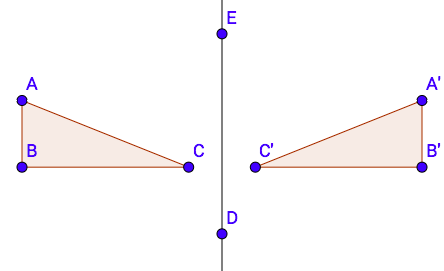• Roughly speaking, a translation takes a figure along a certain vector. For example, in the diagram below, △ABC is now taken to △A’B’C’ by a translation along vector DE.

 Translation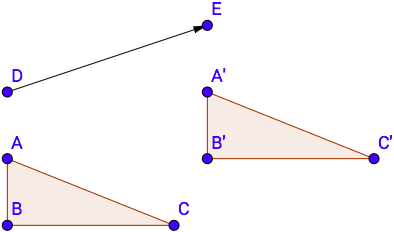• Roughly speaking, a rotation takes a figure around a point (called the center of rotation) by a fixed angle. As a last example, in the diagram below, △ABC is taken to △A’B’C’ by a rotation of 90° clockwise.

 Rotation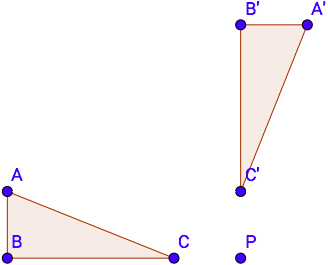• Collectively, these three transformations are sometimes called the basic rigid motions because of the way they move a figure “rigidly” around the plane, preserving the lengths of segments and . (Later in this guide, we’ll discuss a non-rigid transformation: a dilation.)

Memorizing the precise definition of each transformation isn’t important. It’s better if students try each of the transformations and then develop their own definitions as a way of processing the properties of each one. Words like ”flip,” “slide” and “turn” are to be expected at first. However, they don’t capture all of the ideas identified in the standard, and students will soon need to revise them with more detail. All students should be able to say, for example, that a reflection preserves the distances along lines and segments and the measures of angles. They should also notice that the distance between each point on a figure and the line of reflection remains the same when the figure is reflected. It might take several days to work through all three of the rigid motions—focusing on one per day might be a good idea—but it’s time well spent if students are able to define and clearly articulate the properties of each transformation.

### Understanding properties of reflections, translations and rotations

Students should begin their study of congruence with hands-on experiences: trying reflections, translations and rotations for themselves, and describing the properties of figures under these transformations. (8.G.A.1) The best tools for this—clear plastic transparencies (for overhead projectors) or tracing paper—might seem old-fashioned, but they do the job well. There are software packages that allow students to easily experiment with transformations as well (GeoGebra is one popular product, and is available free online) but many teachers find from experience that it’s best for students to have a tactile introduction to all of the rigid motions first.

Let’s take a look at a sample lesson plan to see how introducing one type of transformation might look. This lesson plan deals with reflections, but the basic structure would work just as well for translations or rotations.

#### Grade 8, Module 2, Lesson 4: Example 1

The reflection across a line is defined by using the following example.

• Let 𝐿 be a vertical line, and let 𝑃 and 𝐴 be two points not on 𝐿, as shown below. Also, let 𝑄 be a point on 𝐿. (The black rectangle indicates the border of the paper.)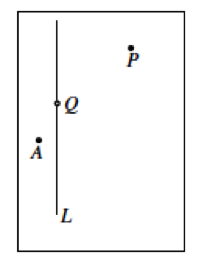• The following is a description of how the reflection moves the points 𝑃, 𝑄, and 𝐴 by making use of the transparency.
• Trace the line 𝐿 and three points onto the transparency exactly, using red. (Be sure to use a transparency that is the same size as the paper.)
• Keeping the paper fixed, flip the transparency across the vertical line (interchanging left and right) while keeping the vertical line and point 𝑄 on top of their black images.
• The position of the red figures on the transparency now represents the reflection of the original figure. Reflection (𝑃) is the point represented by the red dot to the left of 𝐿, Reflection (𝐴) is the red dot to the right of 𝐿, and point Reflection (𝑄) is point 𝑄 itself.
• Note that point is unchanged by the reflection.

HideShow

As you read through, keep in mind that this lesson is part of a unit that uses formal language and notation (including function notation) for transformations. The standards don’t require function notation in Grade 8, and you may not be inclined to introduce formal terms to students on Day 1—that’s okay. What we really want to highlight is the way this lesson introduces students to the concept of a reflection:

• The lesson begins with a hands-on exercise with transparencies. Students can see how the reflection defines the image points, and will probably start to notice the relationship between each point and its image right away. It’s probably better that their first try isn’t on the coordinate plane; the conventions of graphing might distract students from seeing the fundamental properties of reflections.

• Students try a few other exercises. Transparencies are still to be expected at this point. One exercise involves a horizontal line of reflection, and another includes a figure with a vertex on the line of reflection—both good variations for students to see.

• Exercises 3-5 ask some crucial questions that invite students to articulate the key ideas in the standard. They’re asked to compare angle measures and the lengths of segments, and to notice they’re congruent. This helps them formalize those properties in the next step of the lesson. (These ideas might be recorded on an “anchor chart” that hangs in the classroom for the rest of the unit.)

• Further examples have students put new concepts to use.

Expect students to use their transparencies regularly for the first few days. Eventually, they’ll start to develop an intuitive sense of what each transformation will look like, and will rely less and less on transparencies. This “sixth sense” for what the image of a figure will look like is what you’re shooting for, and will allow students to envision solutions to all kinds of problems later on.

### Congruence

Once students have examined the properties of each rigid motion by itself, they can move on to thinking about congruence, and cases where one figure is taken to another by a sequence of rigid motions. (8.G.A.2) Looking back at the standard, we see there are two parts:

1. Students should understand that one figure is congruent to another if one can be taken to the other by a series of rigid motions
2. Students should be able to describe a sequence of rigid motions that takes one figure to another.

The first part means that students should develop a definition of congruence that relies on transformations, and should be able to explain why two figures are congruent based on the properties of the rigid motions. (Note that this is different from the traditional “same shape, same size” definition of congruence.) The second part means pretty much what it says: Students should be able to describe, in some detail, the transformations that take one figure to another. This task is an example:

#### Congruent Triangles

The two triangles in the picture below are congruent: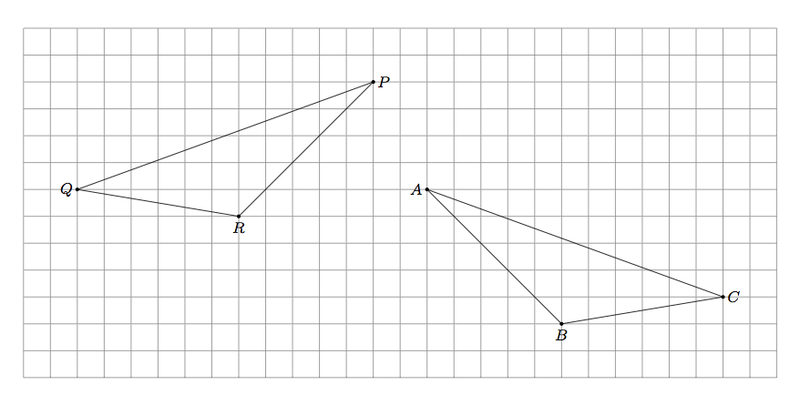1. Give a sequence of rotations, translations, and/or reflections which take △𝑃𝑅𝑄 to △𝐴𝐵𝐶.
2. Is it possible to show the congruence in part (a) using only translations and rotations? Explain.

HideShow

This task is designed to be instructional—the type of thing that kicks off a lesson and leads to new ideas as students move through it. Give it to students after they’re familiar with the rigid motions, and they’ll quickly notice that no single transformation will do it—they’ll have to use more than one. Encourage students to be precise in their explanations. If you see a reflection, where would the line of reflection have to be? If you see a translation, along what vector? A great discussion could arise as students share multiple solutions to the problem.

Once students have tackled the problem, you might introduce the fact that PQR maps exactly to ABC via a translation and a reflection (or other, more complicated sequences), with no gaps or overlaps. Since they’ve used only rigid transformations (which don’t change angle measures or segment lengths) to obtain one figure from the other, we can say they are congruent. From here on out, you want students to use this definition of congruence, and if asked to demonstrate the congruence of two figures, they should do so by way of transformations.

### Dilations

The rigid transformations and their connection to congruence will take some time to solidify. Then it’s time to introduce the concept of similarity, which involves one additional transformation, the dilation. (8.G.A.4) Just as we did above, we’ll begin with an approximate definition, and then clarify with some illustrations. Roughly speaking, then, a dilation is the transformation of a figure (such as a point, line, line segment, polygon, or circle) to another figure by a certain scale factor. In a dilation, the distance between a fixed point (called the center of dilation) and the object being dilated becomes longer or shorter in proportion to the scale factor. The examples below show two dilations of △ABC centered at point P, one with a scale factor greater than 1, and another with a scale factor less than 1.

 Scale factor greater than 1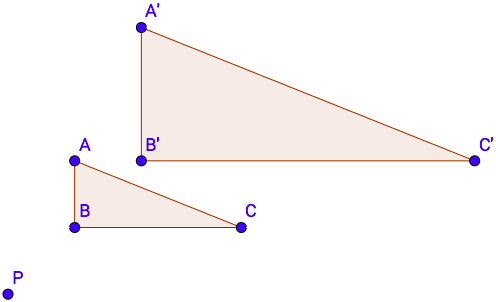Scale factor less than 1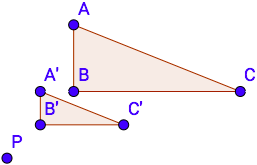As we can see, figures under dilation can be taken to larger or smaller images, depending on the scale factor. This brings up an important point: “Dilation” has a particular mathematical meaning different from its meaning in everyday English (where it simply means an enlargement, not a reduction). It might take students some time to adjust to this new usage of a familiar word, but seeing examples with scale factors of various magnitudes will help the process.

Just as for the rigid motions, students should have some hands-on experiences with dilations, and should use these experiences to develop increasingly precise definitions of dilation. You could use transparencies for such an activity, but the best tools might be a ruler or a compass: Students can measure the distance from each point on a figure to a center point. Then they can try, for example, multiplying those lengths by a scale factor of 2 to produce a dilation. (Likewise, they might try with a scale factor of 1/2.) This lesson plan walks students through an activity that accomplishes this with a compass.

#### Grade 8, Module 3, Lesson 2: Example 1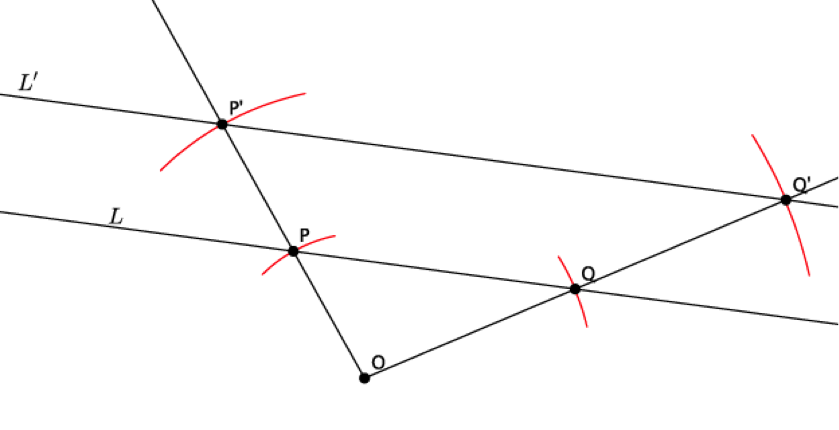Return to your conjecture from before, or look at our class list. Which conjectures were accurate? How do you know?

• Answers may vary depending on conjectures made by the class. Students should identify that the conjecture of a line mapping to a line under a dilation is correct.

What do you think would happen if we selected a different location for the center or for the points 𝑃 and 𝑄?

• Points 𝑂, 𝑃, and 𝑄 are arbitrary points. That means that they could have been anywhere on the plane. For that reason, the results would be the same; that is, the dilation would still produce a line, and the line would be parallel to the original.

Look at the drawing again, and imagine using our transparency to translate the segment 𝑂𝑃 along vector 𝑂𝑃 to segment 𝑃𝑃 ’ and to translate the segment 𝑂𝑄 along vector 𝑂𝑄 to segment 𝑄𝑄 ‘. With that information, can you say anything more about lines 𝐿 and 𝐿’?

• Since 𝑃 and 𝑄 are arbitrary points on line 𝐿, and translations map lines to parallel lines when the vector is not parallel to or part of the original line, we can say that 𝐿 is parallel to 𝐿’.

HideShow

Again, you could use any tools you have available for an activity like this. Students could use rulers to measure the distances from the center of dilation, or they could use transparencies to trace distances. The result is that they develop an intuition for how dilations work and can respond to a variety of follow-up questions. For example, how does changing the scale factor affect the image of a figure? How does moving the center of dilation affect the image of a figure? What would happen if the center of dilation is within, on, or outside of the figure? What would a dilation by a scale factor of 1 produce? Any of these might launch an interesting discussion and help students better understand how dilations behave under various conditions.

### Similarity

Once they have an intuitive sense for how dilations work, students are able to define similarity and describe a series of similarity transformations. (8.G.A.4) Just as with congruence, the goal here is twofold; students should:

1. Understand similarity in terms of rigid motions and dilations.
2. Describe a sequence of similarity transformations.

A task like this one might be a good way to get started:

#### Are They Similar?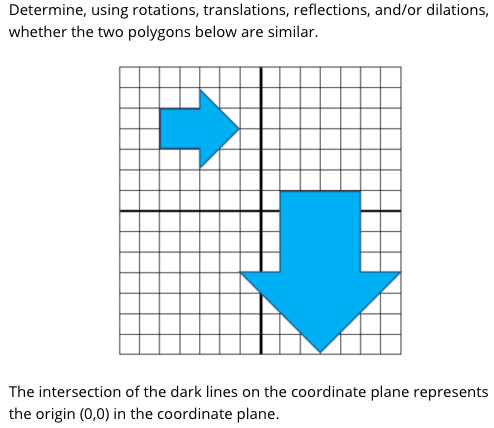You might use this task exactly as is, or you might use this as an instructional task to introduce the idea of similarity through rigid motions and dilations. In that case, we might simplify the question a bit: “Could you map the small arrow to the large one using rigid motions and dilations? Explain your answer.” There are multiple ways of doing this, as shown in the solutions section of the task, and this is an opportunity for rich discussion. Once several methods have been established, you can explain to students that they’ve just shown these figures to be similar because we were able to take one figure to the other via reflections, translations, rotations and dilations. This becomes the definition of similarity. (Note that this is different from the “same shape, different size” definition of similarity that students may be familiar with. It’s not that the two figures are related by a series of transformations and happen to be similar; rather, they’re similar because they’re related by a series of transformations. In other words, once we’ve established a transformational sequence, we’ve established similarity.)

Congruent, similar or both?

It’s important for students to realize that congruence and similarity aren’t mutually exclusive; it isn’t the case that two figures are one but never the other. In fact, if two figures are congruent, they are also similar. To understand why, remember the definition of similarity: One figure is similar to another if it can be obtained by a sequence of reflections, translations, rotations and dilations. Any of the congruence transformations we examined above would also fit this definition—the two figures involved would be congruent but would also meet the criteria for similarity.

### Moving to the coordinate plane

Students’ initial encounters with each transformation (including dilations) need not occur on the coordinate plane. In fact, introducing each transformation in a “synthetic” context (without the use of coordinates, as in the examples shared so far) often allows students to focus on the properties of the transformations themselves without worrying about the conventions of the coordinate plane. It’s also a good reminder that the universe of transformations is much more than just the subset we can describe with integer coordinates. (Students also tend to develop “rules” for performing transformations on the coordinate plane—like “switch the x and y-values” for a 180-degree rotation—that shouldn’t really be the emphasis of early instruction. Recall how our introductory lesson plan on rotations didn’t involve any coordinate grids.)

At some point, though, students should be able to work with transformations on the coordinate plane and clearly describe the effects of certain transformations using coordinates. (8.G.A.3) In essence, this standard is about taking everything students have learned about transformations generally and applying it to problems on the coordinate plane. The variety of problems implied here is tremendous, and presenting an exhaustive sampling just isn’t possible. But let’s take a look at one task as an example of how students make the jump onto the coordinate plane:

#### Point Reflection

The point in the 𝑥-𝑦 plane with coordinates (1000,2012) is reflected across the line 𝑦 = 2000. What are the coordinates of the reflected point?

Again, this is only one example, but let’s examine it closely. In addition to having a solid grasp of the properties of transformations and a basic understanding of the coordinate plane, students also need to be familiar with the equations of horizontal lines. (If you’re not there yet in your year, that’s okay; students could still do a similar task involving a reflection across the x-axis.) Assuming they have all that, they can start solving. It won’t take long to realize that this problem is resistant to brute force; even if a 2,000 x 2,000-square sheet of graph paper did exist, it would take forever to plot the point involved and reflect it. Instead, they’ll have to apply some ideas about reflections: notably, that the distance between a point and the line of reflection is equal to the distance between its image and the line of reflection. Two strategies you might have students try are:

• Have them create a rough sketch of the coordinate plane, or part of the plane, to help them visualize the situation. Then they’ll be able to see that (1000, 2012) is 12 units above y = 2000, so the reflected point will be 12 units below y = 2000. Moreover, the reflection won’t move the image left or right at all, so the x-coordinate of both points will be the same. These two clues should allow them to put the solution together.

• Ask them to think of a simpler case, such as reflecting the point (1, 10) over the line y = 8. Then have them relate the solution to the simpler problem back to the original question. The numbers will be a bit different, but the process will be the same.

In both cases, students are taking a flexible understanding of how transformations work and applying that to specific points and distances on the coordinate plane.

### Special cases of congruence: Transversals and triangles

At first glance, the last standard in cluster 8.G.A might seem out of place. How did we get from transformations to transversals? And what does any of this have to do with the angles of a triangle? These ideas—transformations, transversals, and triangles—have been viewed and taught as separate ideas for a long time. But now, they’re all understood as related. (8.G.A.5) To see how transformations are related to transversals, let’s take a look at this lesson:

#### Grade 8, Module 2, Lesson 12: Exploratory Challenge 2

In the figure below,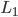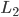, and 𝑚 is a transversal. Use a protractor to measure angles 1–8. List the angles that are equal in measure.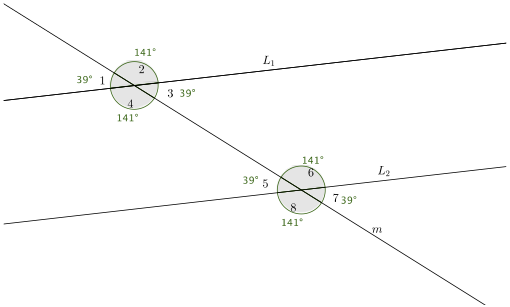∠1 = ∠3 = ∠5 = ∠7 = and ∠2 = ∠4 = ∠6 = ∠8

a. What did you notice about the measures of ∠1 and ∠5? Why do you think this is so? (Use your transparency if needed.)

∠𝟏 and ∠𝟓 are equal in measure. We can translate ∠𝟏 along a vector on line 𝒎 so that the vertex of ∠𝟏 maps onto the vertex of ∠𝟓. Translations are angle-preserving, so the two angles will coincide.

b. What did you notice about the measures of ∠3 and ∠7? Why do you think this is so? (Use your transparency if needed.) Are there any other pairs of angles with this same relationship? If so, list them.

∠𝟑 and ∠𝟕 are equal in measure. We can translate ∠𝟑 along a vector on line 𝒎 so that the vertex of ∠𝟑 maps onto the vertex of ∠𝟕. Translations are angle-preserving, so the two angles will coincide. Other pairs of angles with this same relationship are ∠𝟒 and ∠𝟖 and ∠𝟐 and ∠𝟔.

c. What did you notice about the measures of ∠4 and ∠6? Why do you think this is so? (Use your transparency if needed.) Is there another pair of angles with this same relationship?

The measures of ∠𝟒 and ∠𝟔 are equal. A rotation of 𝟏𝟖𝟎° around a center would map ∠𝟒 to ∠𝟔. Rotations are angle-preserving, so we know that ∠𝟒 and ∠𝟔 are equal. ∠𝟑 and ∠𝟓 have the same relationship.

HideShow

As we can see, students are using a protractor to measure and identify relationships among angles. Then they’re returning to a familiar tool (transparencies) from their work with transformations to understand why these relationships exist. The discussion that follows is particularly interesting: Students should notice how corresponding angles are related by translation of one angle to another. Likewise, alternate interior angles are related by rotation of one angle 180 degrees to another. This is, in fact, the mathematical basis for ideas that were once often given to middle school students as postulates only (“alternate interior angles are congruent,” etc.). After spending time exploring the reasoning behind these angle relationships, students are more likely to remember them and build upon their knowledge in the future.

In the past, another idea often taught in isolation and given to students as a postulate was the sum of a triangle’s interior angles. But after students understand the angles formed by transversals in light of transformations, they’re able to explain where this idea comes from. To see how, let’s look at this task.

#### Triangle’s Interior Angles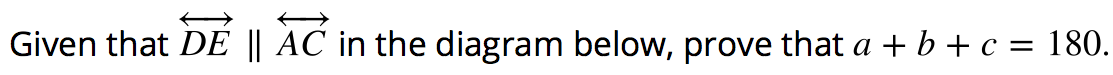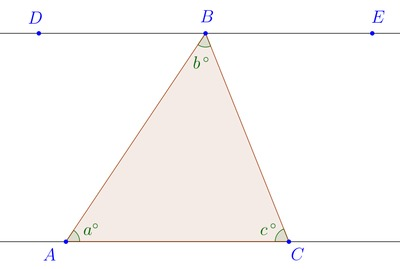Explain why this result holds for any triangle, not just the one displayed above.

One final idea students are prepared to explain by way of transformations is the angle-angle criterion for triangle similarity. This task takes students through a series of questions designed to lead them from a specific case to a more general proposition.

#### Similar Triangles II

Triangles 𝐴𝐵𝐶 and 𝑃𝑄𝑅 below share two pairs of congruent angles as marked: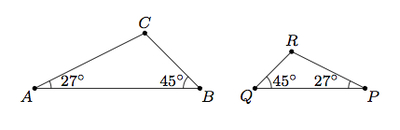1. Explain, using dilations, translations, reflections, and/or rotations, why △𝑃𝑄𝑅 is similar to △𝐴𝐵𝐶.
1. Are angles 𝐶 and 𝑅 congruent?

1. Can you show the similarity in part a without using a reflection? What about without using a dilation? Explain.

1. Suppose 𝐷𝐸𝐹 and 𝐾𝐿𝑀 are two triangles with 𝑚(∠𝐷)=𝑚(∠𝐾) and 𝑚(∠𝐸)=𝑚(∠𝐿). Are triangles 𝐷𝐸𝐹 and 𝐾𝐿𝑀 similar?

HideShow

Parts (a), (b) and (c) of this task rely on students’ understandings of similarity transformations. Then Part (d) asks them, in the same manner as the two previous tasks, to generalize. Given a “general” pair of triangles with two pairs of congruent angles, can they show similarity? Again, students not accustomed to explaining their thinking might need some scaffolding. They might benefit from some possible diagrams of DEF and KLM, in order to see that the exact transformations involved aren’t as important as the fact that some sequence of rigid motions and dilations will take one triangle to the other. One or two sentence starters might also be helpful. As with any of these tasks, the goal isn’t to strip away the essential reasoning required, but to give students another way to see what they’re being asked to think about and explain.

## The Pythagorean Theorem

The other big idea making its debut in Grade 8 geometry is the Pythagorean Theorem. The standards involving the Pythagorean Theorem are also part of the major work of the grade. Given the equations students will encounter when solving problems with the Pythagorean Theorem, such as c2 = 25 and a2 = 17, it might make sense to teach these standards after students are comfortable with square and cube roots (8.EE.A.2) and work with irrational numbers. (8.NS.A.1) (More on the relationships between standards in Part 2 of this guide.) No matter how you choose to sequence your instruction, however, it’s important to realize that a number of high school standards depend on knowing the Pythagorean Theorem, so students should definitely get this content in Grade 8.

The standards involving the Pythagorean Theorem are grouped into another cluster (called 8.G.B, since it’s the second cluster of geometry standards in Grade 8). Let’s take a look at what they have to say.

8.G.B | Understand and apply the Pythagorean Theorem.

 8.G.B.6 Explain a proof of the Pythagorean Theorem and its converse. 8.G.B.7 Apply the Pythagorean Theorem to determine unknown side lengths in right triangles in real-world and mathematical problems in two and three dimensions. 8.G.B.8 Apply the Pythagorean Theorem to find the distance between two points in a coordinate system.

Again, the order of the standards doesn’t indicate the order in which they have to be taught. But in this case, it makes sense to begin with a proof of the Pythagorean Theorem (8.G.B.6), and then move on to solving various sorts of problems (8.G.B.7 and 8.G.B.8).

Before we begin talking about the standards in this cluster, let’s put two important ideas front and center.

• The Pythagorean Theorem says: If a triangle is a right triangle, the lengths of the legs are a and b, and the length of the hypotenuse is c, then a2 + b2 = c2.
• The converse of the Pythagorean Theorem is also true: If a triangle has sides of lengths a, b and c, and a2 + b2 = c2, then that triangle is a right triangle.

In the past, this topic was often introduced to students by giving them a formula (the one named above: a2 + b2 = c2) and explaining what the variables stand for. Instruction then revolved around relatively simple problems in which students were given two side lengths on a right triangle and had to find the third. That sort of problem-solving still happens, but the Standards begin by asking students to explain a proof of the Pythagorean Theorem and its converse. (8.G.B.6) In so doing, they learn two important things:

• The Pythagorean Theorem describes a relationship among sides of a right triangle (not just a formula). When students understand the nature of the relationship and are able to express it verbally (as well as in algebraic terms), they’re set up to apply it in a wider range of situations, and to extend their learning later on.
• The converse of the Pythagorean Theorem is just as useful as the Theorem itself. Students learn that they can use the Theorem not just when they know they have a right triangle, but also when they need to establish that a triangle is a right triangle.

So, where does the Pythagorean Theorem come from? There are actually many different proofs, some of them more complicated than others. These different proofs rely on different techniques and provide different reasons for the Pythagorean Theorem to be true. This lesson uses a “square within a square” proof involving area, beginning like this:

#### Grade 8, Module 2, Lesson 15: Discussion

The first proof of the Pythagorean theorem requires knowledge of some basic facts about geometry.

1. Congruent triangles have equal areas.
2. All corresponding parts of congruent triangles are congruent.
3. The triangle sum theorem. (∠ sum of △)
1. For right triangles, the two angles that are not the right angle have a sum of 90°. (∠ sum of rt. △)

What we look at next is what is called a square within a square. The outside square has side lengths (a+b), and the inside square has side lengths c. Our goal is to show that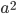+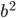=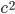To accomplish this goal, we compare the total area of the outside square with the parts it is composed of, that is, the four triangles and the smaller inside square.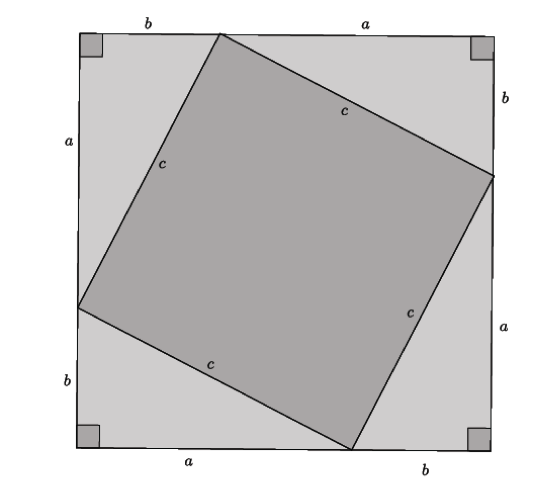HideShow

The rest of the proof, which you can see in the lesson plan, helps students understand that the inner figure is really a square, and then uses an area calculation to derive the formula. If you’re going to use this lesson plan, think about the amount of support you want to give—perhaps students can make it through some of the proof themselves, and finish with some guidance from you, or maybe they need more structure. (One warning: The algebra involved in that last calculation involves multiplying two binomials, which isn’t a skill required until high school. You may need to help students apply the distributive property in this new way.) Either way, they’re understanding that the Pythagorean Theorem involves an aspect of area, which is useful for interpreting certain types of application problems.

## Applying the Pythagorean Theorem to solve problems

Once students understand the origin of the Pythagorean Theorem, it’s onto problem-solving. (8.G.B.7) Initially, students might take on problems involving whole numbers only, in order to focus on interpreting a situation in terms of a right triangle and making accurate calculations. (The lesson plan above includes some examples of these introductory-level problems.) This task requires a bit more interpretation.

#### Running on the Football Field

During the 2005 Divisional Playoff game between The Denver Broncos and The New England Patriots, Bronco player Champ Bailey intercepted Tom Brady around the goal line (see the circled B). He ran the ball nearly all the way to other goal line. Ben Watson of the New England Patriots (see the circled W) chased after Champ and tracked him down just before the other goal line.

In the image below, each hash mark is equal to one yard: note too that the field is 53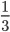yards wide.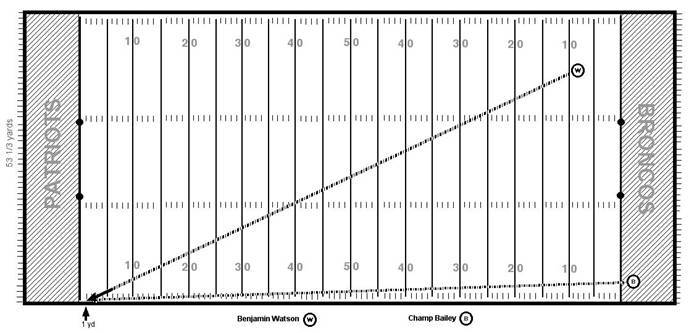1. How can you use the diagram and the Pythagorean Theorem to find approximately how many yards Ben Watson ran to track down Champ Bailey?

1. Use the Pythagorean Theorem to find approximately how many yards Watson ran in this play.

1. Which player ran further during this play? By approximately how many more yards?

HideShow

Students might need to read the problem a few times to understand what each part of the diagram represents. And as you can see, some estimation is in order. Given that the entire field is 100 yards long and 53 1/2 yards wide, about how long are the sides of this near-triangle? Once students are able to see the right triangle and its dimensions, this problem becomes very manageable.

### Finding distances on the coordinate plane

Students should also use the Pythagorean Theorem to find distances on the coordinate plane. (8.G.B.8) This is really a specific variant of the problems we just discussed; students learn to interpret two points on the coordinate plane as defining the hypotenuse of a right triangle. This problem comes from an introductory lesson on this idea.

#### Grade 8, Module 7, Lesson 17: Example 1

What is the distance between the two points on the coordinate plane? Round your answer to the tenths place.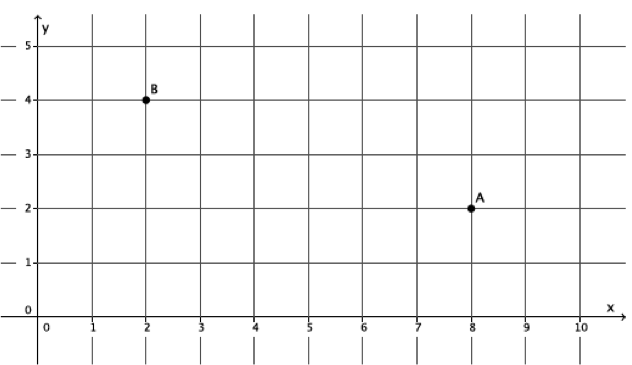Before coming to this problem, students encounter a sequence of questions in which they find the lengths of some horizontal and vertical segments, understanding that the length of a segment on the coordinate plane is just the number of squares it covers. Moreover, they see it’s not possible to find the distance of a diagonal segment by counting squares. This particular problem is then posed as a challenge, and students have some time to work on it. (Chances are that when given in the context of a unit on the Pythagorean Theorem, a number of students will think to apply their new learning here. Ideally, students should be able to explain to their peers how to draw the right triangle from these points and solve for the length in question.)

After a problem like this, students should practice finding the distance between points in different quadrants of the coordinate plane, as well as the distance between two points given as ordered pairs and not plotted on the plane. (If you’re interested in a task that can be used to help them do this, check out “Finding the Distance between Points” from Illustrative Mathematics. The commentary and solutions give some ideas about how students can move from finding the distance between two specific, numbered points to the general case of any two coordinate pairs.)

## The role of Mathematical Practices

The Standards don’t just include knowledge and skills; they also recognize the need for students to engage in certain important practices of mathematical thinking and communication. These “Mathematical Practices” have their own set of standards, which contain the same basic objectives for Grades K-12.3 You can read the full text of the Standards for Mathematical Practice here. You can read the full text of the Standards for Mathematical Practice here. You can read the full text of the Standards for Mathematical Practice here. (The idea is that students should cultivate the same habits of mind in increasingly sophisticated ways over the years.) But rather than being “just another thing” for teachers to incorporate into their classes, the practices are ways to help students arrive at the deep conceptual understandings required in each grade. In other words, the Practices help students get the content. The table below contains a few examples of how the Mathematical Practices might help students understand and apply geometry concepts in Grade 8.

 Opportunities for Mathematical Practices: Teacher actions: Work with transformations offers students the opportunity to construct viable arguments and critique the reasoning of others. (MP.3) For example, students can informally argue the congruence or similarity of two figures using sequences of transformations. After establishing the concept of congruence (and later, the concept of similarity), you can ask students to explain whether two figures are congruent and/or similar. Is there more than one way to establish similarity? Can two figures be both congruent and similar? While students may require transparencies or other tools in order to make their arguments, answering these sorts of questions helps students deepen their understanding of congruence and similarity, and prepares them for the formal reasoning required in high school geometry. Students can make use of structure (MP.7) as they derive new ideas (the relationships among angles formed by transversals, the sum of a triangle’s interior angles, etc.) from earlier notions of transformations. For example, students should see a transversal as a familiar structure (a translation vector) which explains the congruence between a pair of corresponding angles. You can introduce the angles formed by transversals as an application of transformations, asking students to explain the relationships they’re seeing in terms of rigid motions. (And in a related way, you can introduce the sum of a triangle’s interior angles as a special case of transversals.) Eventually, students should come to see the components of geometry problems as “clues” about which prior understandings might give them insight into a solution. Students can reason abstractly and quantitatively (MP.2) when they solve problems with the Pythagorean Theorem, which abstracts a comparison between the three quantities in a right triangle. With practice, students should learn to view problem situations in terms of right triangles, as well as to construe their solutions in the contexts of problems. When approaching new kinds of problem situations in class, have students do the work of identifying right triangles, assigning variables to their side lengths and interpreting the meaning of solutions. If c = 17, for example, what does this mean in the context of the problem? In order to cement their understanding further, have students explain how they made each of these decisions.

Podcast clip: Importance of the Mathematical Practices with Andrew Chen and Peter Coe (start 30:33, end 43:39)

# Part 2: How do congruence, similarity and the Pythagorean Theorem relate to other parts of Grade 8?

## Transformations: Connection to Expressions & Equations

Transformations are crucial to understanding one other piece major work for Grade 8: slope.4 The idea that standards relate strongly to one another is known as coherence, and is a distinctive feature of the Common Core State Standards for Math. If you’re interested in exploring more of the connections between standards, you might want to try the Student Achievement Partners Coherence Map web app. The idea that standards relate strongly to one another is known as coherence, and is a distinctive feature of the Common Core State Standards for Math. If you’re interested in exploring more of the connections between standards, you might want to try the Student Achievement Partners Coherence Map web app. The idea that standards relate strongly to one another is known as coherence, and is a distinctive feature of the Common Core State Standards for Math. If you’re interested in exploring more of the connections between standards, you might want to try the Student Achievement Partners Coherence Map web app. Students first encounter slope through graphs of proportional relationships, understanding it as the unit rate of the relationship. (This is a concept first developed in Grade 7.) Why, though, is the slope of a line constant? This is often a question that gets swept under the rug. One way of understanding is through an application of similarity: Every line on the coordinate plane implies any number of similar “slope triangles” with proportional sides. (8.EE.B.6) This task shows one example of how students might approach this concept.

#### Slopes between Points on a Line

The slope between two points is calculated by finding the change in y-values and dividing by the change in x-values. For example, the slope between the points (7, -15) and (-8, 22) can be computed as follows:

• The difference in the y-values is −15 − 22 = −37.
• The difference in the x-values is 7 − (−8) = 15.
• Dividing these two differences, we find that the slope is −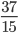.

Eva, Carl, and Maria are computing the slope between pairs of points on the line shown below.Eva finds the slope between the points (0,0) and (3,2). Carl finds the slope between the points (3,2) and (6,4). Maria finds the slope between the points (3,2) and (9,6). They have each drawn a triangle to help with their calculations (shown below).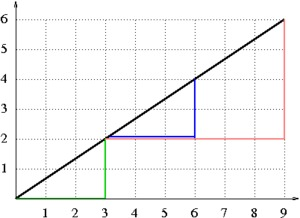1. Which student has drawn which triangle? Finish the slope calculation for each student. How can the differences in the x- and y-values be interpreted geometrically in the pictures they have drawn?

1. Consider any two points (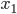,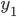) and (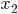,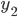) on the line shown above. Draw a triangle like the triangles drawn by Eva, Carl, and Maria. What is the slope between these two points? Why should this slope be the same as the slopes calculated by the three students?

HideShow

As noted in the solution, the triangles in this task are designed so that students can easily define them as similar using a series of rigid motions and dilations. Since any one triangle on the line can be taken to another larger or smaller triangle by a translation and a dilation, they must be similar. Thus, students are able to demonstrate that the slope between any two points on any given line will be the same. (This is a more deductive and mathematically sound way of understanding the slope of a line than simply observing that the slope between a few pairs of points on one particular line happens to be the same.)

## Pythagorean Theorem: Connection to Expressions & Equations and The Number System

The Pythagorean Theorem is tightly connected to work in the Number System (NS) and Expressions & Equations (EE) domains. The EE standards are part of the major work of Grade 8, while the NS standards are designated as “supporting” work, since they have the potential to strengthen and extend major topics.

In Grade 8, the NS standards introduce students to irrational numbers (numbers like √2, which can’t be expressed as fractions), (8.NS.A.1) and the EE standards expose students to simple equations like x2 = 8 and y3 = 27, which involve solving with square and cube roots. (8.EE.A.2) Since application of the Pythagorean Theorem naturally gives rise to equations of this type, problem-solving in the context of right triangles represents a convergence of two standards. Take, for example, our problem from standard 8.G.B.8 above: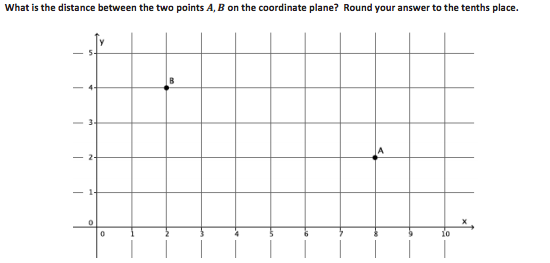When students solve, they obtain the equation 22 + 62 = c2, and their solution looks something like this:

22 + 62 = c2

4 + 36 = c2

40 = c2

√40 = c

Based on work with standard 8.NS.A.1, a student in Grade 8 should be able to say that √40 is a number between 6 and 7 because 40 is between 36 (62) and 49 (72). From there, they can reason that it’s less than 6.5 because 40 is closer to 36 than 49, and use successive approximations to find that it’s about 6.3. Looking back at the problem, which involves distances of 2 units and 6 units for the legs, this is a reasonable length to expect for the hypotenuse.

# Part 3: Where did congruence, similarity and the Pythagorean Theorem come from, and where are they going?

## Where do congruence and similarity come from?

While congruence and similarity begin in Grade 8, more fundamental concepts in geometry should be underway throughout the elementary and middle grades. As early as Kindergarten, they begin thinking about length and classifying shapes. (K.G.A.2) Grade 4 turns out to be critical for work with congruence and similarity: The concept of angle measure is defined, (4.MD.C.5) as are points, lines, segments and rays. (4.G.A.1) In Grade 5, students learn about the coordinate plane and plot points in the first quadrant. (5.G.A.1) Then, in Grade 6, students expand their knowledge of the number system to include negative numbers, thereby revealing the entire coordinate plane. (6.NS.C.8) Work on the coordinate plane continues in Grade 7, (7.RP.A.2) and students solve problems involving scale drawings. (7.G.A.1) While these problems don’t require a formal understanding of similarity in terms of transformations, they give students an opportunity to work with pairs of similar figures and scale factors.

Podcast clip: Importance of Coherence with Andrew Chen and Peter Coe (start 9:34, end 26:19)

 Grade 4: Angles Monique looked at the clock at the beginning of class and at the end of class. How many degrees did the minute hand turn from the beginning of class until the end?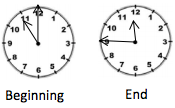(Source: Grade 4, Module 4, Lesson 8 (teacher version) from EngageNY.org of the New York State Education Department is licensed under CC BY-NC-SA 3.0.) ➔ Problems like this help solidify the concept of an angle as a fraction of a turn. Students in Grade 4 should also learn to distinguish angle measure from length or area—the size of the clock can change, but the amount that the hands turn remains the same.

 Grades 5-6: The coordinate plane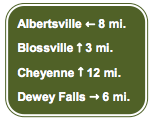Four friends are touring on motorcycles. They come to an intersection of two roads; the road they are on continues straight, and the other is perpendicular to it. The sign at the intersection shows the distances to several towns. Draw a map/diagram of the roads, and use it and the information on the sign to answer the following questions: What is the distance between Albertsville and Dewey Falls? What is the distance between Blossville and Cheyenne? On the coordinate plane, what represents the intersection of the two roads? (Source: Grade 6, Module 3, Lesson 19 (teacher version) from EngageNY.org of the New York State Education Department is licensed under CC BY-NC-SA 3.0.) ➔ In Grade 5, students only plot points in the first quadrant of the coordinate plane. In Grade 6, as they develop an understanding of negative numbers and absolute value, they’re able to explore all four quadrants. This problem invites students to draw the complete x-y coordinate system and think about distances along the axes.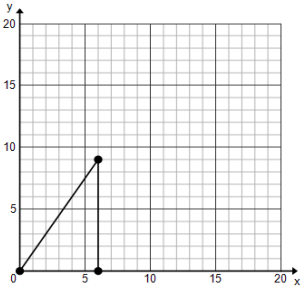a. If the original lengths are multiplied by 2, what are the new coordinates?

b. Use the table to organize lengths (the vertical and horizontal legs).

 Width Height Actual Picture (in units) New Picture (in units)

c. Is the new picture a reduction or an enlargement?

d. What is the constant of proportionality?

While the focus in Grade 7 is more on the proportionality of similar figures, they expose students to scale factor and dilations in an informal way. After problems like this, students should bring an understanding of these ideas into their work with similarity transformations in Grade 8.

## Where does the Pythagorean Theorem come from?

The Pythagorean Theorem is new in Grade 8 as well. While it doesn’t rely on a long progression of learning, some basic prerequisites do apply:

• Students will need to be comfortable with exponential notation from Grade 6 in order to make sense of the algebraic formula. (6.EE.A.1)
• As noted above, students need experience solving equations from Grades 6, 7 and 8 in order to solve problems with the Pythagorean Theorem. (6.EE.B.7, 7.EE.B.4, 8.EE.A.2)
• Much like transformations, solid understanding of the Pythagorean Theorem relies on elementary geometry concepts like length and angle measure. (4.G.A.1)

## Suggestions for students who are behind

If, going into a unit on congruence and similarity, you know your students don’t have a solid grasp of the ideas named above (or haven’t encountered them at all), what can you do? It’s not practical (or even desirable) to re-teach everything students should have learned in Grades 4-7; there’s plenty of new material to learn in Grade 8, so the focus needs to be on grade-level standards. At the same time, there are strategic ways of wrapping up “unfinished learning” from prior grades within a unit on congruence. Here are a few ideas for adapting your instruction to bridge the gaps.

• If a significant number of students don’t fully understand angles or other elementary concepts, you could plan a lesson or two focused on these ideas before you begin teaching grade-level content. (Two Grade 4 lessons, one on angle measurement and the other on distinguishing length from angle measure, might be helpful here.) If you think that an entire lesson is too much, you could plan a brief “mini-lesson,” or use some problems involving these ideas as warm-ups for your first few lessons on congruence.
• If a significant number of students don’t have experience with the coordinate plane or aren’t yet able to accurately plot points, you could plan a lesson or series of warm-ups on this idea before starting work with transformations on the coordinate plane. (This Grade 6 lesson on drawing polygons on the coordinate plane might be a good source of problems for this type of lesson.)
• If a significant number of students aren’t able to fluently identify and describe geometric figures (points, lines, line segments, etc.), you could plan a mini-lesson or a series of warm-ups to review them during the first few days of a unit involving transformations. (This Grade 4 lesson, which introduces the terms just mentioned, contains a simple introduction to these ideas, and the problems that follow the lesson might also be useful.)

## Where are these concepts going?

Congruence and similarity have long been essential ideas in high school, and as explained above, transformations are now central in understanding these ideas. One of the first experiences students will have in high school geometry is to develop precise definitions of the rigid motions. (G-CO.A.4) Using transformations allows students to establish congruence criteria for triangles (G-CO.B.8) and gives them a tool to use when proving theorems. (G-CO.C) Once students understand congruence, they’re ready to solve problems involving congruent figures, (G.SRT.B.5) explore relationships within triangles, (G-C.A.2) and prove statements using coordinates. (G-GPE.B.4) Down the road, they’ll encounter more specialized applications of congruence; Cavalieri's Principle, for example, depends on knowing what it means for cross-sections of two different figures to be congruent. (G-GMD.A.1) Similarity involves a similar process and leads into the study of trigonometry.G-SRT.C.9 One last progression shows how work in Grade 8 leads into high school.

 Grade 8: Angles In the following picture, we have two pairs of triangles. In each pair, triangle ABC can be traced onto a transparency and mapped onto triangle A’B’C′. Which basic rigid motion, or sequence of, would map one triangle onto the other? Scenario 1: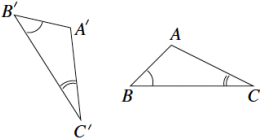Scenario 2: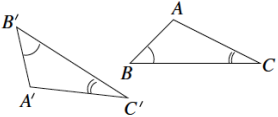(Source: Grade 8, Module 2, Lesson 10 (teacher version) from EngageNY.org of the New York State Education Department is licensed under CC BY-NC-SA 3.0.) ➔ In this Grade 8 problem, similar to others in this guide, students determine the sequence of rigid motions necessary to take one figure to another. Knowing the relationship between rigid motions and congruence will help them develop congruence criteria for triangles, prove congruence theorems, and solve problems involving congruent figures in high school.

 High school: Congruence (G-CO) In triangles ABC and ABD below, we are given that angle BAC is congruent to angle BAD and angle ABC is congruent to angle ABD. Show that the reflection of the plane about line AB maps triangle ABD to triangle ABC.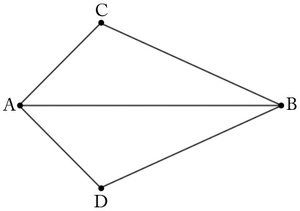(Source: “Why Does ASA Work?” by Illustrative Mathematics is licensed under CC BY 4.0.) ➔ In this problem, students use reflections to establish the ASA criterion for triangle congruence. Later, they’ll be able to use this criterion in the context of proofs and problem solving.

 High school: Similarity (G-SRT) Alicia has two triangles, ABC and PQR, whose corresponding sides are proportional as pictured below: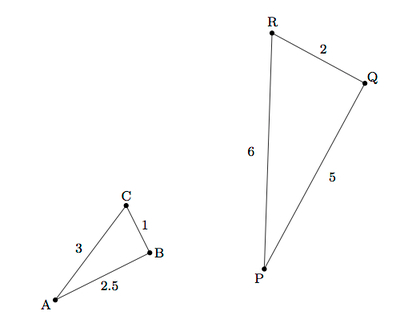Alicia says: I wonder if they are similar because I don't have any information about the angles? What is the answer to Alicia's question? Explain. (Source: “Similar Triangles” by Illustrative Mathematics is licensed under CC BY 4.0.) ➔ In this problem, students use transformations to explain the meaning of similarity for triangles (equality of angles and proportionality of sides). This is a major stepping stone to developing similarity criteria for triangles and proving theorems about similarity.

Student Achievement Partners: Focus in Grade 8

Hung-Hsi Wu: Teaching Geometry According to the Common Core Standards

EngageNY: Grade 8, Module 2 Materials (Congruence)

EngageNY: Grade 8, Module 3 Materials (Similarity)

EngageNY: Grade 8, Module 7 Materials (Pythagorean Theorem)

# Endnotes

 In this series, major clusters are denoted by a . For more information on the major work of Grade 8, see the Student Achievement Partners guide Focus in Grade 8.

 If you’re interested in precise definitions of each of these transformations, see pages 65-76 of Hung-Hsi Wu’s Teaching Geometry According to the Common Core Standards.

 You can read the full text of the Standards for Mathematical Practice here.

 The idea that standards relate strongly to one another is known as coherence, and is a distinctive feature of the Common Core State Standards for Math. If you’re interested in exploring more of the connections between standards, you might want to try the Student Achievement Partners Coherence Map web app.

# FAQs

## 1. What is a Content Guide?

Our goal in creating the Content Guides has been to provide busy teachers with a practical and easy-to-read resource on what the grade-level math standards are saying, along with examples of instructional materials that support conceptual understanding, problem-solving, and procedural skill and fluency for students.

It’s important to note that content guides are not meant to serve as a curriculum (or any kind of student-facing document), a guide or source material for test-preparation activities, or any kind of teacher evaluation tool.

## 2. What’s in a Content Guide?

Each Content Guide is focused on a specific group of standards. Most Content Guides follow the same three-part structure:

• Part 1 makes clear the student skills and understandings described by this group of standards. This section illustrates the standards using multiple student tasks from freely available online sources. Teachers can use or adapt these tasks for their students.
• Part 2 explains how this group of standards is connected to other standards in the same grade. We highlight how these connections have implications for planning and teaching, and how this within-grade coherence can increase access for students. Part 2 also includes multiple student tasks from freely available online sources.

• Part 3 traces selected progressions of learning leading to grade-level content discussed in the specific Content Guide. This discussion segues into a series of concrete and practical suggestions for how teachers can leverage the progressions to teach students who may not be prepared for grade-level mathematics. Finally, Part 3 traces the progression to content in higher grades.

## 3. How can I use the Content Guides?

Teachers who have read our Content Guides say they see benefits for all educators. Here are some suggestions for how different educators might use them.

Teachers can use the Mathematics Content Guides to:

• Increase or refresh their knowledge of the standards and the expectations for what students should know by the end of the year.
• Adapt lessons and units using appropriate pre-requisites to support students who are behind grade-level.
• Gain access to the best available OER for math to use for introducing and/or reinforcing concepts
• Ensure their curriculum and/or units:
• Focus on the major work of the grade and the appropriate depth of each standard.
• Target the appropriate aspects of rigor—procedural skill and fluency, modeling and application, and conceptual understanding described by the standards.
• Help students make coherent connections within and across grades.
• Create or revise their lessons and questioning to focus on important concepts in the standards.

Instructional coaches and school leaders can use the Mathematics Content Guides to:

• Refresh or increase their knowledge of the standards and the expectations for what students should know by the end of the year.
• Develop and communicate consistent expectations for lesson planning and instruction aligned to the standards.
• Provide a reference when planning and/or discussing instruction with teachers.
• Gain insight into what instruction and student work should look like in order to meet the demands of the standards.
• Develop and design content and standards-driven professional development sessions/workshops.
• Foster content rich, standards-based discussions among staff and build staff knowledge.
• Develop and/or revise school improvement plans in order to support and incorporate content and practice-based teaching and learning.

## 4. Why the Content Guides?

The transition to higher standards has led teachers all over the country to make significant changes in their planning and instruction, but only one-third of teachers feel they are prepared to help their students pass the more rigorous standards-aligned assessments (Kane et.al., 2016). This is to be expected because the new high standards are a significant departure from prior standards. The standards require a deeper level of understanding of the math content they teach; a different progression of what students need to learn by which grade; as well as different pedagogy that emphasizes student conceptual understanding, problem solving and procedural fluency in equal intensity.

The support for teachers to bring high standards to their classrooms, however, has lagged behind. Research shows that teacher training in the U.S. is currently insufficient in preparing teachers to teach the demanding new standards (Center for Research in Mathematics and Science Education, 2010). And though some resources exist that “unpack” the standards, few, if any, explain and illustrate the standards. “Unpacking” the standards one by one can also result in a disjointed presentation that neglects the structure and coherence of the standards. In creating the Content Guides, we aimed to provide busy teachers with a practical, easy-to-read resource on their grade-specific standards and how to help all students learn them. There is ample empirical evidence that when teachers have both strong knowledge of the math content that they teach, and the pedagogical knowledge to help students master that content knowledge, their students learn more (Baumert et. al., 2010; Hill, Rowan and Ball, 2005; Rockoff et. al., 2008). With the Content Guides in hand, we hope that teachers will find more success in helping their students make progress toward college- and career-readiness.

## 5. What is the relationship between the Content Guides and the Progressions?

The Progressions documents describe the grade-to-grade development of understanding of mathematics. These were informed by research on children’s cognitive development as well as the logical structure of mathematics. The Progressions explain why standards are sequenced the way they are. The Content Guides often highlight key ideas from the Progressions, but do not add new standards or change the expectations of what students should know and be able to do; they aim to explain and illustrate a group of standards at a time using freely available online sources. While the OER tasks and lessons in the Content Guides are one way to meet the grade-level standards, they are not the only means for doing so.

## 6. How were the resources selected?

We selected sample tasks and lessons from freely available online sources such as EngageNY, Illustrative Mathematics and Student Achievement Partners to illustrate the Standards. These sources are chosen because they are fully aligned to the new high standards based on national review of K-12 curricula or are created by organizations led by the writers of the new high standards. In addition, because they are open educational resources (OER), they are freely accessible for all uses. All UnboundEd materials are also OER, as part of our commitment to make high-quality, highly aligned content available to all educators.